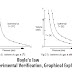## Boyle's law.

The volume of a given amount of gas is inversely proportional to the pressure of the gas at a constant temperature.

'k' is the proportionality constant and 'n' is the number of moles of gas. The value of 'k' is different for the different amounts of the same gas. It also depends upon the pressure and quantity of the gas. According to equation (1), Boyle's law has got another definition.

Definition:

The product of pressure and volume of a fixed amount of a gas is a constant quantity at a constant temperature. Let the initial pressure and initial volumes are P1 and V1. The final pressure and final volumes are P2 and V2.

Since, P1V1    =   k       and   P2V  =  k
So,  P1V1       =   P2V2     ............... (2)

### Experimental Verification of Boyle's Law.

Boyle's law can be verified by working on the apparatus shown in Fig (3. 1).
Take a gas in a cylinder with a movable piston. This is also attached to a manometer to record the pressure.

The volume "V1" the given quantity of gas at pressure P1 = 1 atm is reduced in proportion to the increase in pressure when the temperature is kept constant. When the piston is pressed twice the pressure becomes two atmospheres. When this piston is pressed three times, then the pressure becomes three atmospheres. If the initial volume at two atmospheres is 1dm3, then it becomes 0.5 dm3' and then 0.33 dm3 respectively with increasing pressures. The value of the product of pressure and volume remains the same at the same temperature.

Hence,
P1V1    =   2  atm  x   1 dm3   =   2 dm3  atm.  = k
P1V1    =   4  atm  x   0.5 dm3   =   2 dm3  atm.  = k
P1V1    =   6  atm  x   0.33 dm3   =   2 dm3  atm.  = k

The product of pressure and volume has the units of dm atmosphere.

### Graphical Explanation of Boyle's Law.

In order to explain Boyle's law, we can plot a graph between the pressure of the gas on the x-axis and the volume of the gas at the y-axis at a constant temperature. The amount of gas also remains constant.
For this purpose, consider a particular amount of gas at 0°C. The gas is enclosed in a piston-cylinder arrangement. When the pressure of the gas is changed its volume also changes. The graph appears in the form of a curve. Fig (3.2).

This curve at fixed temperature and quantity of a gas is called isotherm of the gas.

#### Isotherms at various temperatures.

If the temperature of the above-mentioned gas is maintained at 25°C and again the isotherm is plotted then it goes away from the axis. Fig (3.3).

Reason:

The reason is that at the higher temperature the volume is increased at the same values of pressures. In this way, the points of the curve move away from the axis.
Similarly, if the temperature is increased up to 40°C, then the isotherm becomes further away from the axis.
Boyle's law | Experimental Verification, Graphical Explanation |Reviewed by Genuine Chemistry on July 25, 2020 Rating: 5